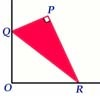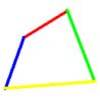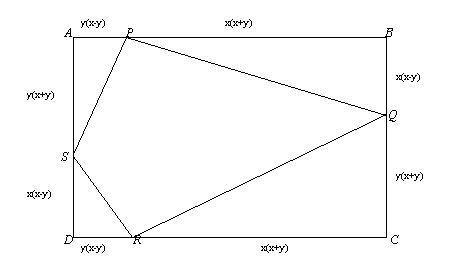You may also likeSet Square

A triangle PQR, right angled at P, slides on a horizontal floor with Q and R in contact with perpendicular walls. What is the locus of P?Biggest Bendy

Four rods are hinged at their ends to form a quadrilateral. How can you maximise its area?Strange Rectangle 2

Find the exact values of some trig. ratios from this rectangle in which a cyclic quadrilateral cuts off four right angled triangles.

Strange Rectangle

Age 16 to 18Challenge LevelShow that, for any values of $x$ and $y$ where $x> y$,

1. the angle $SPQ$ is always the same and find this angle
2. the angle $SRQ$ is always the same and find this angle
3. the quadrilateral $SPQR$ is cyclic
4. the angle $PQR$ is always the same and find this angle
5. the angle $PSR$ is always the same and find this angle.